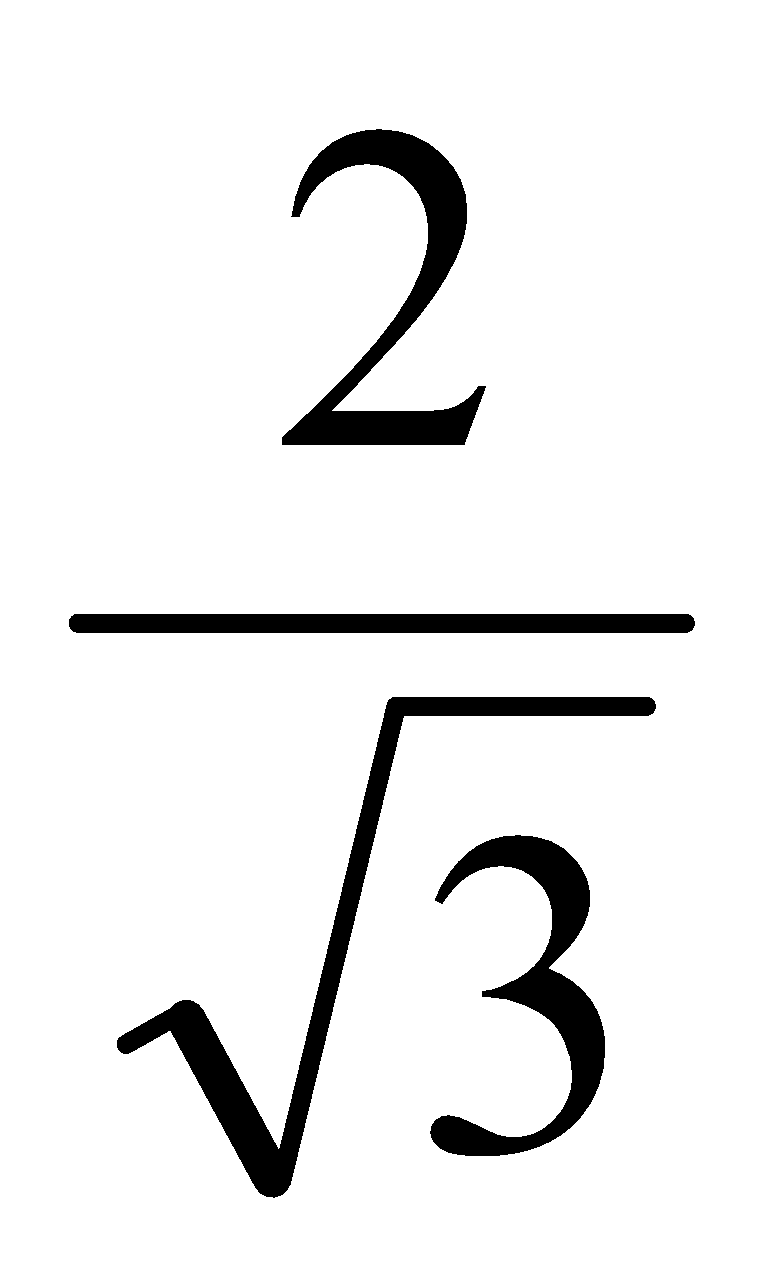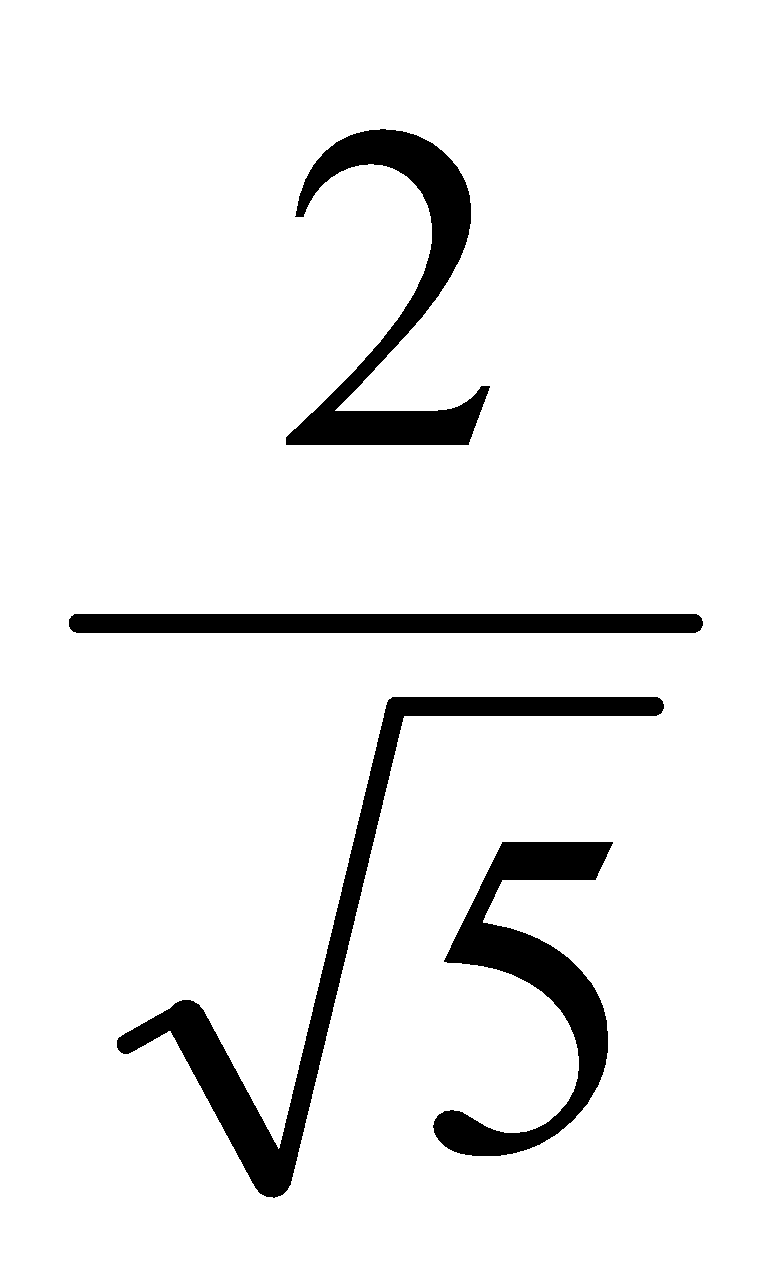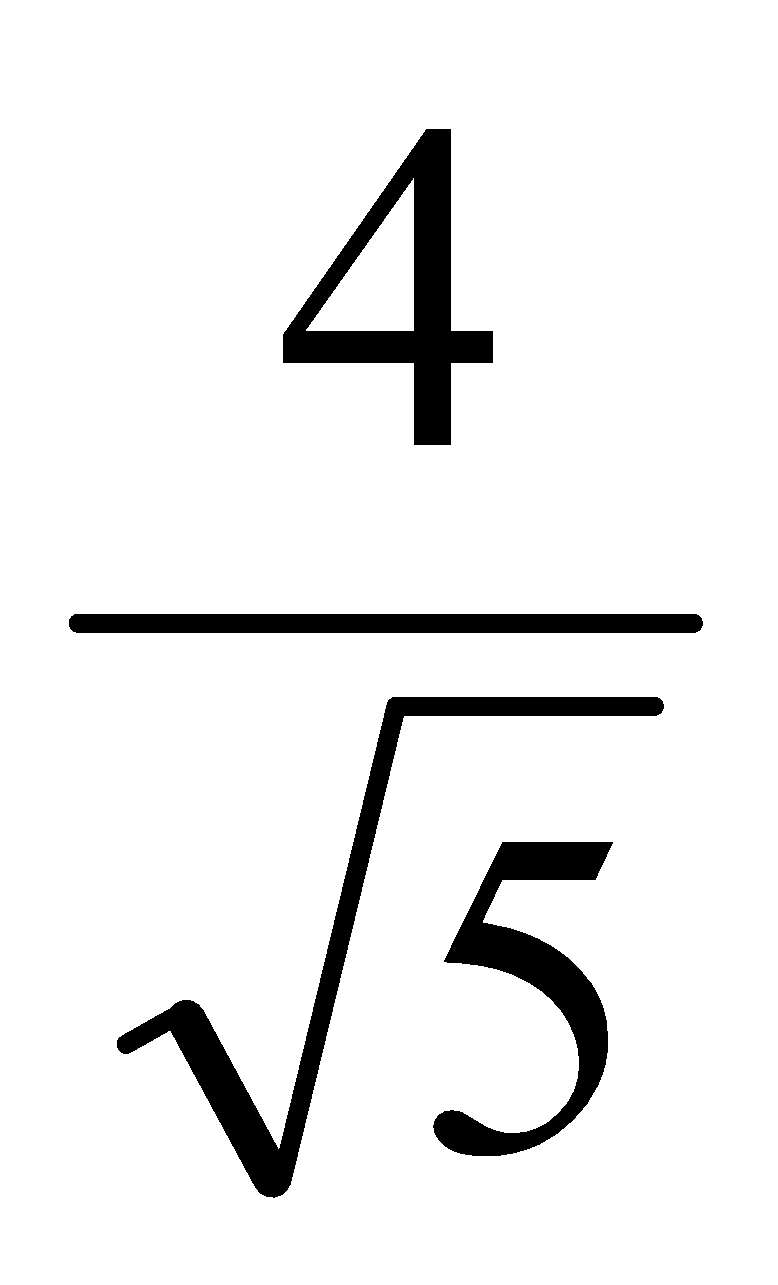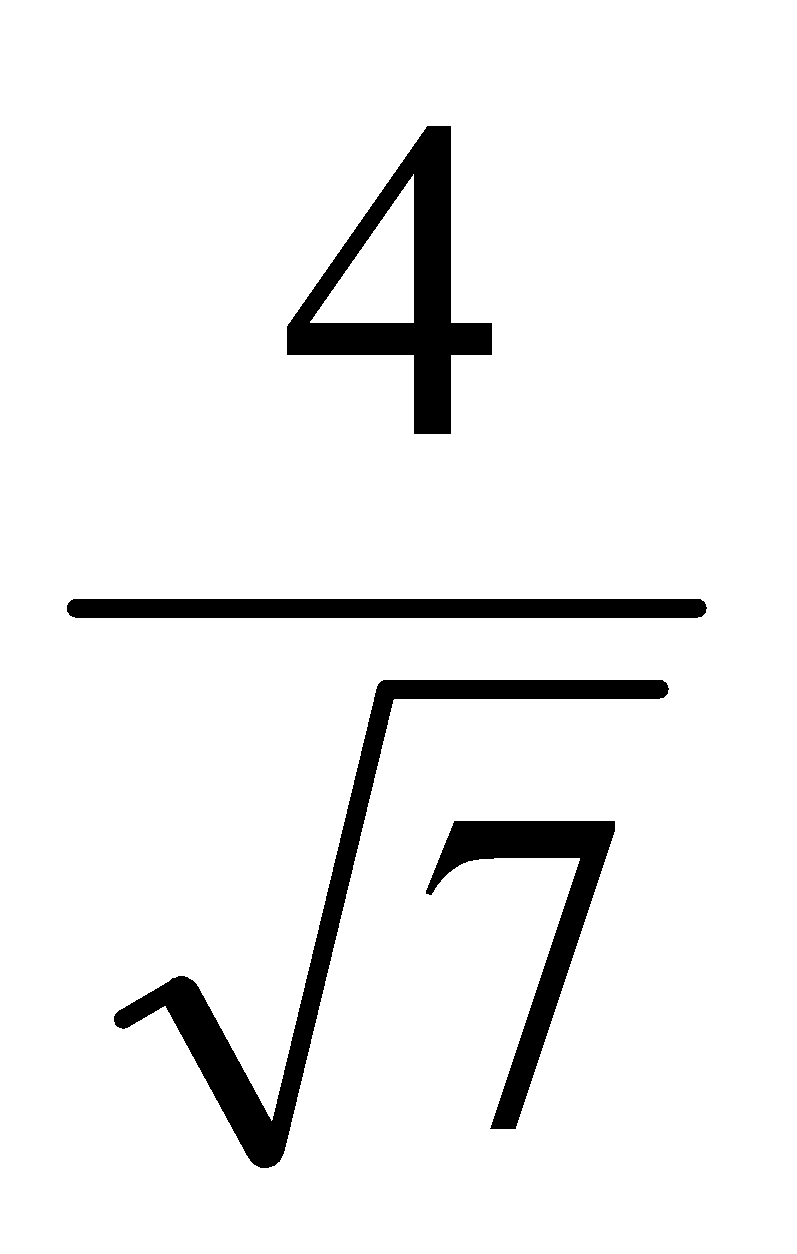Question of Exercise 1

# Question An opaque sphere of radius a is just immersed in a transparent liquid as shown in figure. A point source is placed on the vertical diameter of the sphere at a distance a/2 from the top of the sphere. One ray originating from the point source after refraction from the air liquid interface forms tangent to the sphere. The angle of refraction for that particular ray is 30°. The refractive index of the liquid is

Option 1Option 2Option 3Option 4Calculate the stopping potential Vs2

A: 4.47

B: 3.16

C: 2.76

D: 5.28

Solution:

Explanation::

Therefore, option A is correct that is 4.47 V.

State and explain Coulombs law in electrostatics

Solution:

Explanation

• Coulomb’s law in electrostatic states that a charge at rest q1 which applies a force F on the other charge q2, which is also at

rest both the charge are separated by the distance r. The force is directly proportional to the product of bothe of these charges

and inversely proportional to the square of the same distance which is separated both the charges

.A cube of side b has a charge q at each of its vertices

Determine the potential and electric field due to this charge array at the centre of the cube.

Solution:

Explanation

First make the diagram of the cube of side b, that is shown belowThe clear sky appears blue because

A: blue light gets absorbed in the atmosphere.

B: ultraviolet radiations are absorbed in the atmosphere.

C: violet and blue lights get scattered more than lights of all other colours by the atmosphere.

D: light of all other colours is scattered more than the violet and blue colour lights by the atmosphere.

Solution:

Explanation

• Rayleigh scattering of sunlight causes the clear sky to seem blue.
• The sun's blue light is scattered more by the molecules in the air than it is by red light.
• The size of the dust particles and the wavelength of the light affect light scattering.
• The quantity of scattering is inversely proportional to the wavelength multiplied by the fourth power.
• The sky appears blue because violet and blue sunlight are dispersed more than all other colours by the atmosphere.

The correct option is C i.e violet and blue lights get scattered more than lights of all other colours by the atmosphere.

The image formed by convex lens in a simple microscope is

A: Virtual and erect

B: Real and inverted

C: Of same size as the object

D: None of these

Solution:

Explanation

The image formed by the convex lens in a simple microscope is virtual, erect and magnified.

This can be clearly seen with the help of the ray diagram: